# Creating an Amortization loan or mortgage schedule using Excel 2007 and Excel 2010

## Introduction

Hi and welcome to my latest hub on Excel. This is Part Two of my hub on creating a mortgage calculator. In Part One we created a basic mortgage calculator using a number of functions from both Excel 2007 and Excel 2010 (PMT, PPMT and IPMT to calculate repayment amount, principal paid and interest paid respectively as well as ABS which was used to convert a number from a negative to a positive). That hub can be found here:

http://robbiecwilson.hubpages.com/hub/Using-the-PMT-PPMT-IMPT-and-ABS-functions-to-create-a-Mortgage-Calculator-in-Excel-2007-and-Excel-2010

Today in Part Two, I will expand on that hub and using the basic mortgage (or loan) calculator we will create an amortization schedule. An amortization schedule basically illustrates the balance of your loan or mortgage after each payment as well as indicating how much principal and interest you pay each time you make a payment. In addition, it will illustrate the effect of any overpayments you make on your remaining loan or mortgage.

We will once again use the PMT, PPMT and IPMT functions to calculate the value of the loan or mortgage over time. In addition, we will use the IFERROR function to suppress any #NUM errors we may see if the duration of the mortgage or loan is shortened. I have a hub that investigates the IFERROR and IF functions in more detail which can also be found here:

http://robbiecwilson.hubpages.com/hub/Using-the-IF-and-IFERROR-functions-as-well-as-the-logical-functions-AND-OR-and-NOT-in-Excel-2007-and-Excel-2010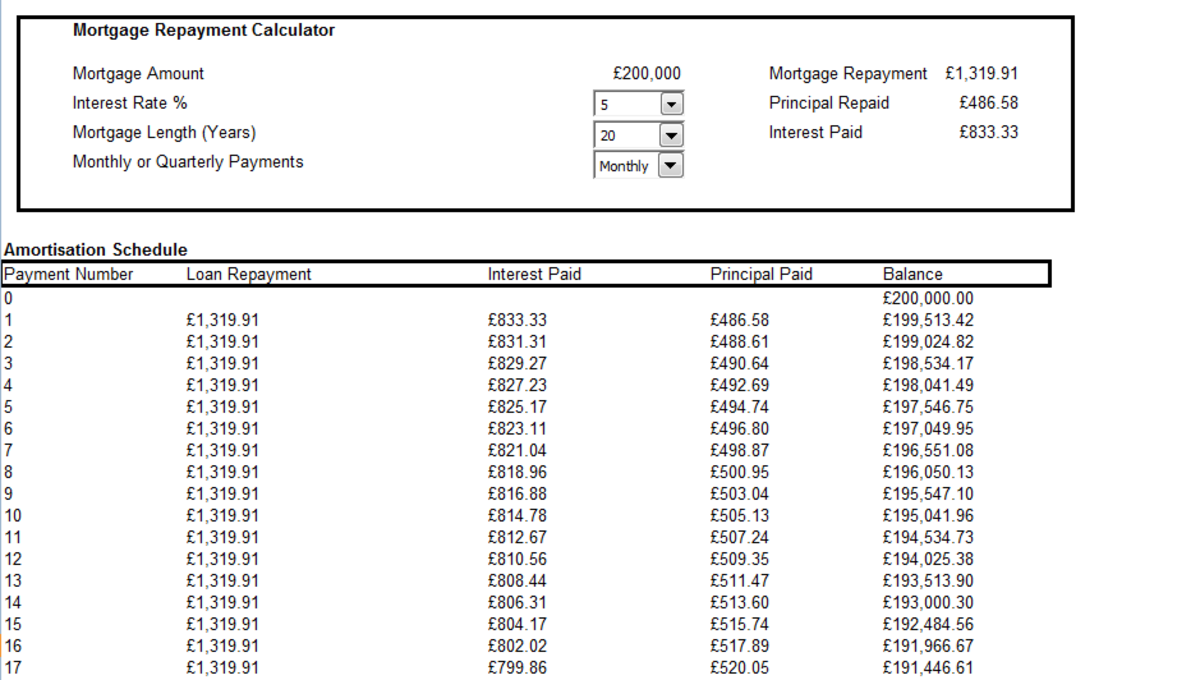Example of an Amortization schedule created in Excel 2007 and Excel 2010 used to calculate the balance of a loan or mortgage after each payment as well as indicating how much principal and interest is paid in each payment.http://robbiecwilson.hubpages.com/

## Manually creating an Amortization schedule in Excel 2007 and Excel 2010

Before using the automatic template, let us look at creating the schedule manually. That way, not only do we gain an understanding of how the template works, but we also gain knowledge of how to use three functions in Excel 2007 and Excel 2010 that we may be able to use in other situations in the future.

To begin, we have to link our amortization schedule with the mortgage calculator that we made in Part One. The data will feed directly from the calculator to our amortization feed.

The first line which is Payment 0 (or the beginning of the schedule) is simply the balance of the mortgage or loan. The formula is simply:

=F16

So if the user of the calculator changes the loan or mortgage amount this is picked up by the schedule. So the formula becomes

=\$I\$16

Note: the \$ is vitally important as it makes it an absolute reference so that Excel does not change the I or the 16 when you copy it. This is particularly important for the subsequent formulas.

## Calculating the interest paid on a mortgage using Excel 2007 and Excel 2010

The next formula calculates the interest portion of the repayment using the IPMT function.

The formula is as follows (I have used a figure so that I can highlight the variable that does not use a \$):

=IPMT(\$R\$2/\$M\$2,A30,\$M\$5*\$M\$2,-\$F\$16)Using the IPMT function to calculate the interest paid on a mortgage or loan in Excel 2007 and Excel 2010.http://robbiecwilson.hubpages.com/

The formula in detail is (I have used bold to make it easier to see):

=IPMT(Interest Rate% / Number of repayments per year, the payment number, the number of repayments per year * the number of years, - the mortgage amount)

Note: There are a number of important things to note in this formula.

Scroll to Continue

The Interest Rate must have a %

The payment number must not have a \$ for the row number

The mortgage amount must have a so that the result is positive

When creating your own formula you must use a \$ everywhere I did in mine otherwise your results will not be accurate.

## Calculating the Principal paid on a mortgage using Excel 2007 and Excel 2010

The calculation for the amount of principal paid uses the PPMT function. The syntax of the formula is identical to the syntax used above for IPMT.

=PPMT(\$R\$2/\$M\$2,A30,\$M\$2*\$M\$5,-\$F\$16)Using the PPMT function to calculate the principal paid on a mortgage or loan in Excel 2007 and Excel 2010.http://robbiecwilson.hubpages.com/

Once again the same care must be taken with the \$ in the formula, if they are missed out then the formula will fail to produce accurate results.

## Calculating the balance of a mortgage using Excel 2007 and Excel 2010

The final formula we need to add is the formula for calculating the balance after the repayment is made.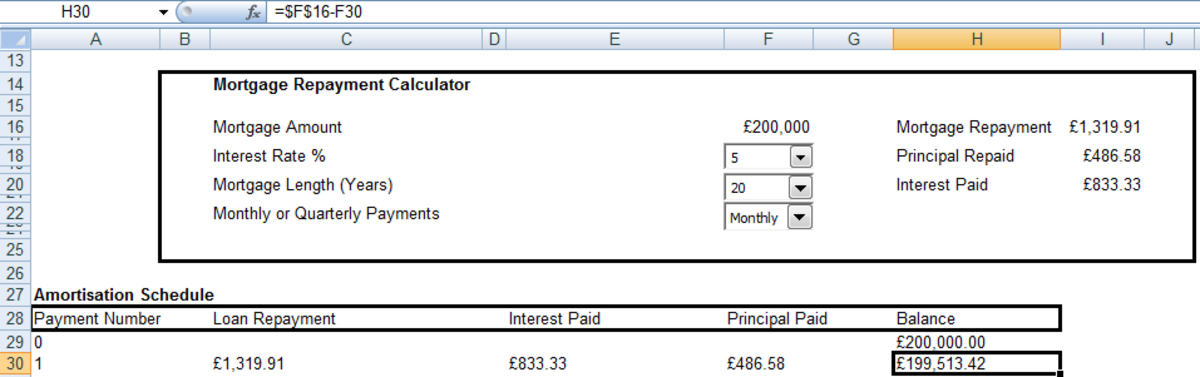Calculating the balance of the loan or mortgage in an Amortization schedule created in Excel 2007 and Excel 2010. http://robbiecwilson.hubpages.com/

The formula is:

=\$F\$16-F30

Note: As above, the \$ are crucial to allow this formula to work correctly.

## Creating the complete Amortisation schedule using Excel 2007 and Excel 2010

Now that we have that crucial second row completed, we need to fill in the remaining rows.

To fill in the Payment number column,

• Select the cell in the second row and in the Payment number column (A30 in my example)
• Click on the Fill button in the Editing group on the Home tab
• Select Series
• Select Series in Columns
• For Stop value select the number of payments (240 in my example)

You can see how it should look in the below figure.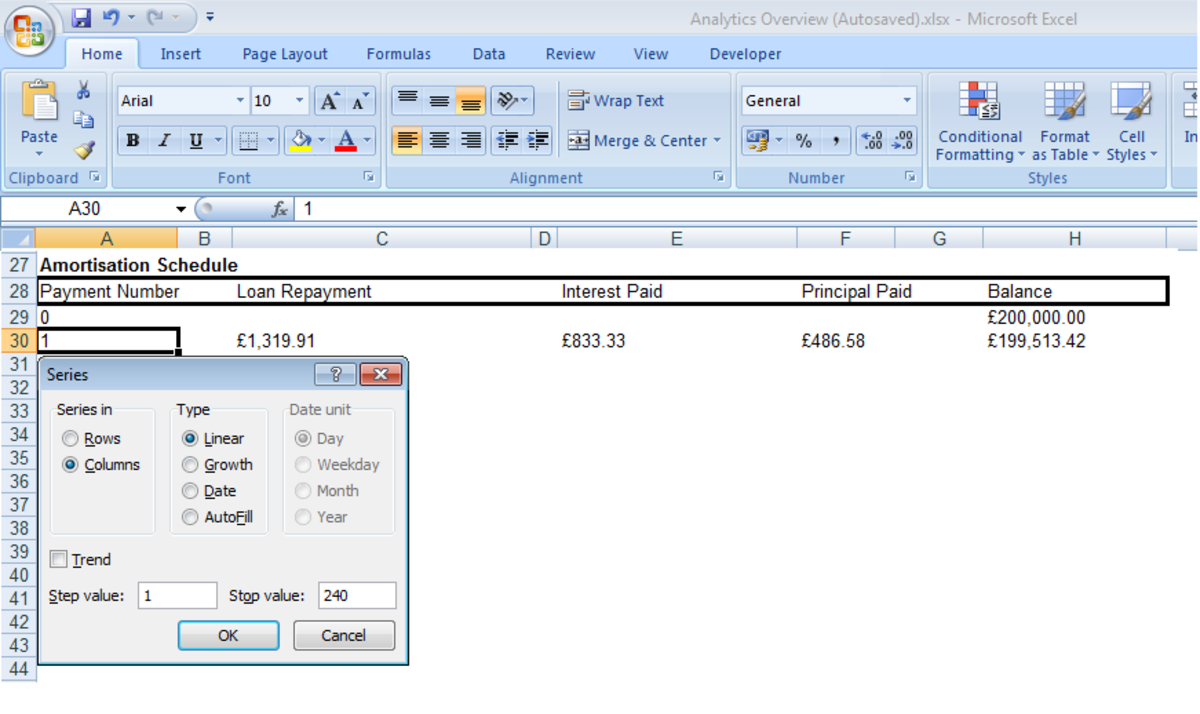Creating a series in a column in Excel 2007 and Excel 2010.http://robbiecwilson.hubpages.com/

To complete the remaining columns, select the row of cells containing your first values for Loan Repayment, Interest Paid, Principal Paid and the Balance and drag it to the last Payment Number row.

## Adjusting the Amortisation schedule created using Excel 2007 and Excel 2010

As the schedule is dynamic, any changes to interest rate or loan or mortgage amount, for example, will be automatically reflected in the schedule.

If you increase or decrease the number of years, simply add or remove rows as you need to fill up the schedule.

To quickly add rows, extend the series as we did above. To populate the remaining columns:

• Select the cells containing our formulas in the last row of numbers
• Double click on the bottom right hand corner (shown by the arrow in the below figure)
• The remaining rows will auto-fill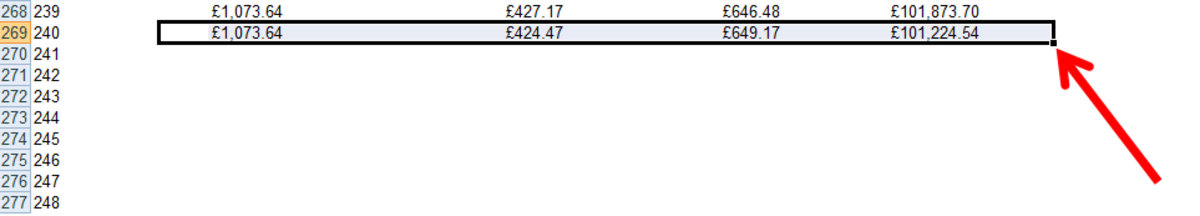How to quickly populate rows in Excel 2007 and Excel 2010.http://robbiecwilson.hubpages.com/

## Removing #NUM errors in the Amortization schedule created using Excel 2007 and Excel 2010

When adjusting the mortgage or loan length to a shorter period, you will notice that you end up with a load of #NUM errors. To resolve this, we need to make a change to the formulas in our spreadsheet. We will use the IFERROR function in Excel to suppress the #NUM errors:

• Click on the first row of formulas in the Interest Paid column

The formula will be similar to

=IPMT(\$R\$2/\$M\$2,A30,\$M\$5*\$M\$2,-\$F\$16)

• Add =IFERROR( to the beginning of the formula and delete the = in front of IPMT
• Add ,” “) at the end of the formula
• The formula becomes:

=IFERROR(IPMT(\$R\$2/\$M\$2,A270,\$M\$5*\$M\$2,-\$F\$16)," ")

• Populate the rest of your spreadsheet using these adjusted formulas

Now, if I scroll down and select one of the cells that used to contain a #NUM, I can see that it has a formula in it but no longer displays an error.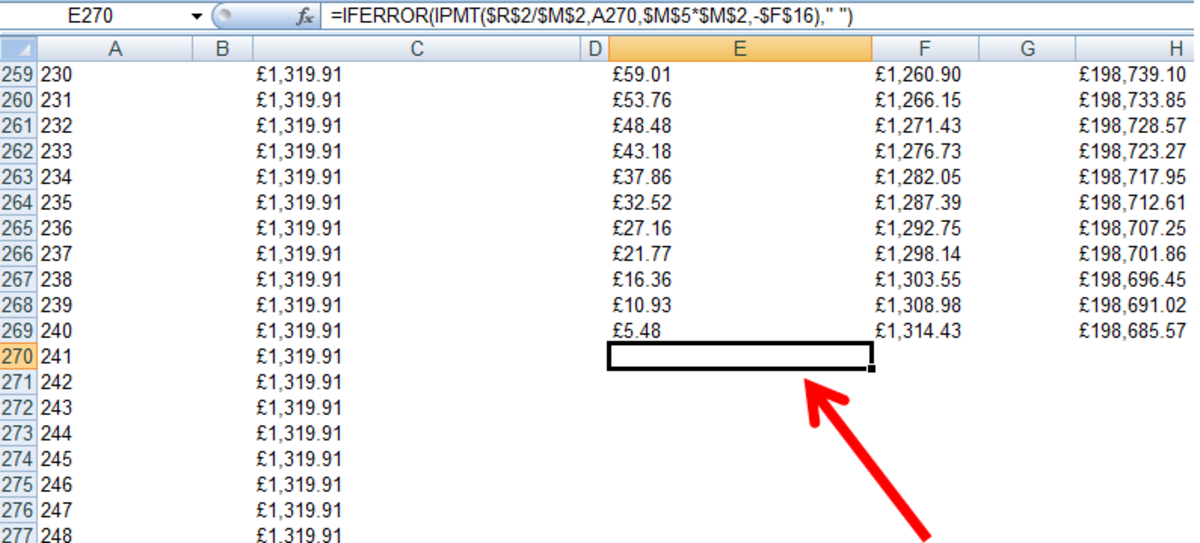Populating rows with formulas with the IFERROR function suppressing errors in Excel 2007 and Excel 2010.http://robbiecwilson.hubpages.com/

## Using Amortization Templates in Excel 2007 and Excel 2010

Now that we understand how to create an amortization schedule manually, it is worth pointing out that you can use a prebuilt template as well. In Excel 2007

• Click the Excel button
• Select New
• Type Amortization into the search window and click the arrow
• Microsoft will search online and return results similar to mine below:
• Your new template will now be available via New on the My Templates tab

For Excel 2010:

• Select the File Menu
• Click New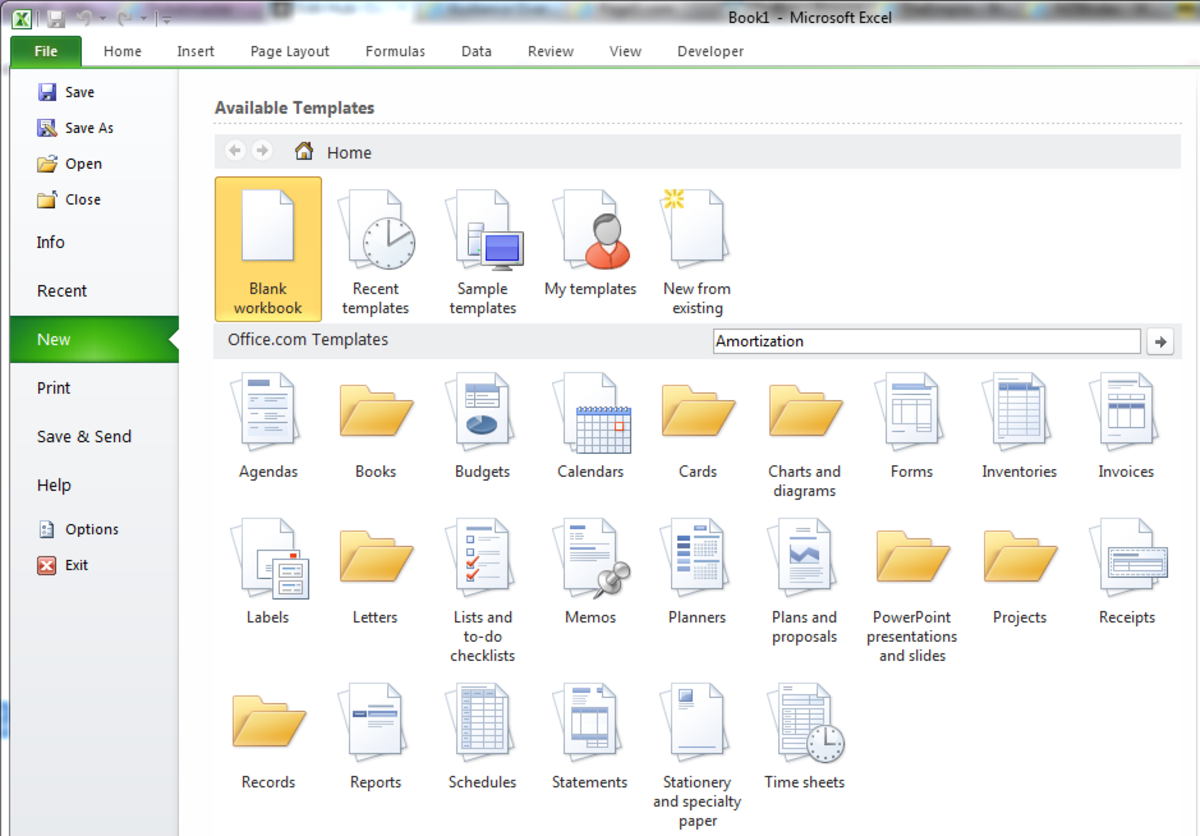Using Office Online to find an Amortization template in Excel 2010.http://robbiecwilson.hubpages.com/
• As with Excel 2007, click Download
• Your new template will be added to your Recent Templates and My Templates via the File menu / New

## Conclusion

In today’s hub, we expanded on the basic mortgage calculator we created in Part One of this series http://robbiecwilson.hubpages.com/hub/Using-the-PMT-PPMT-IMPT-and-ABS-functions-to-create-a-Mortgage-Calculator-in-Excel-2007-and-Excel-2010 and created an amortization schedule.

• Once again, we used the PMT, IPMT and PPMT functions to calculate the repayment amount as well as the portion of the repayments that were interest and principal.
• With the formulas in place, we looked at creating a series to automatically populate the repayment period.
• Finally, we used the IFERROR function to suppress the #NUM errors that you receive when you shorten the period of the loan or mortgage.

I hope that you have found this hub and also Part One useful and informative. Thanks for reading, please feel free to leave a comment below.